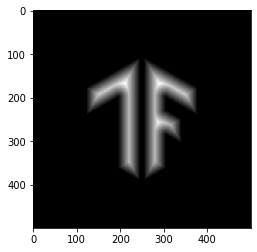Save the date! Google I/O returns May 18-20

# TensorFlow Addons Image: Operations

## Overview

This notebook will demonstrate how to use the some image operations in TensorFlow Addons.

Here is the list of image operations you'll be covering in this example:

# Setup

````pip install -q -U tensorflow-addons`
```
``````import tensorflow as tf
import numpy as np
import tensorflow_addons as tfa
import matplotlib.pyplot as plt
``````

# Prepare and Inspect Images

## Download the images

``````img_path = tf.keras.utils.get_file('tensorflow.png','https://tensorflow.org/images/tf_logo.png')
``````
```Downloading data from https://tensorflow.org/images/tf_logo.png
40960/39781 [==============================] - 0s 3us/step

```

## Inspect the images

### TensorFlow Icon

``````img_raw = tf.io.read_file(img_path)
img = tf.io.decode_image(img_raw)
img = tf.image.convert_image_dtype(img, tf.float32)
img = tf.image.resize(img, [500,500])

plt.title("TensorFlow Logo with shape {}".format(img.shape))
_ = plt.imshow(img)
``````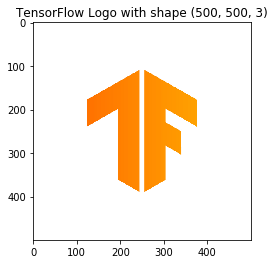### Make a black and white version

``````bw_img = 1.0 - tf.image.rgb_to_grayscale(img)

plt.title("Mask image with shape {}".format(bw_img.shape))
_ = plt.imshow(bw_img[...,0], cmap='gray')
``````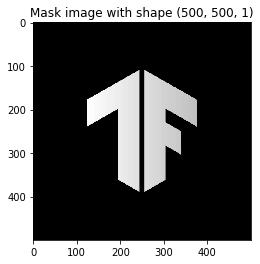# Play with tfa.image

## Mean filtering

Mean filtering is a filtering technique, which is often used to remove noise from an image or signal. The idea is to run through the image pixel by pixel and replacing it with the average values of neighboring pixels.

``````mean = tfa.image.mean_filter2d(img, filter_shape=11)
_ = plt.imshow(mean)
``````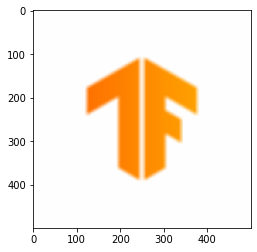## Rotate

This operation rotates the given image by the angle (in radians) input by the user.

``````rotate = tfa.image.rotate(img, tf.constant(np.pi/8))
_ = plt.imshow(rotate)
``````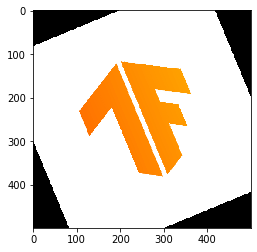## Transform

This operation transforms the given image on the basis of the transform vector given by the user.

``````transform = tfa.image.transform(img, [1.0, 1.0, -250, 0.0, 1.0, 0.0, 0.0, 0.0])
_ = plt.imshow(transform)
``````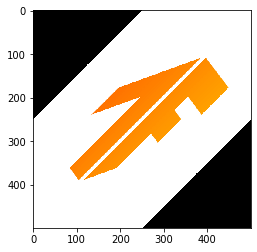## Random HSV in YIQ

This operation changes color scale of a given RGB image to YIQ but here delta hue and saturation values are picked randomly from the given range.

``````delta = 0.5
lower_saturation = 0.1
upper_saturation = 0.9
lower_value = 0.2
upper_value = 0.8
rand_hsvinyiq = tfa.image.random_hsv_in_yiq(img, delta, lower_saturation, upper_saturation, lower_value, upper_value)
_ = plt.imshow(rand_hsvinyiq)
``````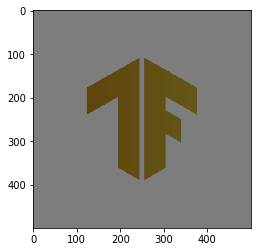## Adjust HSV in YIQ

This operation changes color scale of a given RGB image to YIQ but here instead of choosing randomly, delta hue and saturation values are inputs form the user.

``````delta = 0.5
saturation = 0.3
value = 0.6
adj_hsvinyiq = tfa.image.adjust_hsv_in_yiq(img, delta, saturation, value)
_ = plt.imshow(adj_hsvinyiq)
``````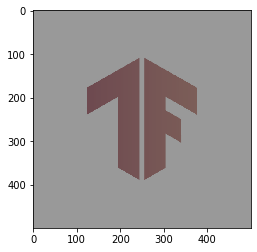## Dense Image Warp

This operation is for non-linear warp of any image specified by the flow field of the offset vector (here used random values for example).

``````input_img = tf.image.convert_image_dtype(tf.expand_dims(img, 0), tf.dtypes.float32)

flow_shape = [1, input_img.shape, input_img.shape, 2]
init_flows = np.float32(np.random.normal(size=flow_shape) * 2.0)
dense_img_warp = tfa.image.dense_image_warp(input_img, init_flows)
dense_img_warp = tf.squeeze(dense_img_warp, 0)
_ = plt.imshow(dense_img_warp)
``````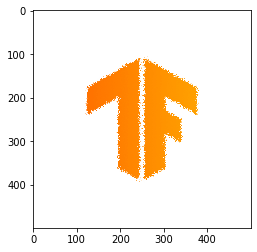## Euclidian Distance Transform

This operation updates the pixel value with the euclidian distance from the foreground pixel to the background one.

• Note : It takes only binary image and results in transformed image. If a different image is given it results in a image with single value
``````gray = tf.image.convert_image_dtype(bw_img,tf.uint8)
# The op expects a batch of images, so add a batch dimension
gray = tf.expand_dims(gray, 0)
eucid = tfa.image.euclidean_dist_transform(gray)
eucid = tf.squeeze(eucid, (0, -1))
_ = plt.imshow(eucid, cmap='gray')
``````